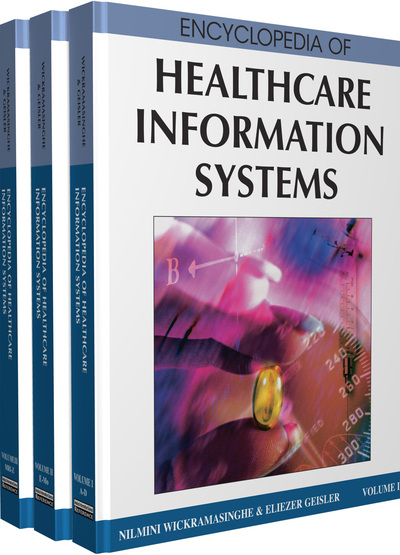Biomedical Signal Compression

Pedro de A. Berger (University of Brasilia, Brazil), Francisco A. de O. Nascimento (University of Brasilia, Brazil), Leonardo R.A.X. de Menezes (University of Brasilia, Brazil), Adson F. da Rocha (University of Brasilia, Brazil) and Joao L.A. Carvalho (University of Southern California, USA)
DOI: 10.4018/978-1-59904-889-5.ch022
Available
\$37.50
No Current Special Offers

Abstract

Digitization of biomedical signals has been used in several areas. Some of these include ambulatory monitoring, phone line transmission, database storage, and several other applications in health and biomedical engineering. These applications have helped in diagnostics, patient care, and remote treatment. One example is the digital transmission of ECG signals, from the patient’s house or ambulance to the hospital. This has been proven useful in cardiac diagnoses. Biomedical signals need to be digitally stored or transmitted with a large number of samples per second, and with a great number of bits per sample, in order to assure the required fidelity of the waveform for visual inspection. Therefore, the use of signal compression techniques is fundamental for cost reduction and technical feasibility of storage and transmission of biomedical signals.

Key Terms in this Chapter

Wavelet Transform: Refers to a transformation where a signal containing N samples is first filtered by a pair of filters that decompose the signal into low and a high frequency bands. Each band is undersampled by a factor of two, that is, each band contains N/2 samples. With the appropriate filter design, this action is reversible.

Delta Coding: Refers to signal compression techniques that store a digital signal as the difference between successive samples. The first sample in the encoded signal is equal to the first sample of the original signal. Subsequent encoded samples are equal to the difference between the current sample and the previous sample of the original signal.

Signal Compression: The reduction of the necessary amount of bits to represent a signal. In many cases, this must be accomplished while preserving the morphological characteristics of the waveform. In theory, signal compression is the process where the redundant information contained in the signal is detected and discarded.

Direct Methods: Encode signals in time domain. These algorithms depend on the morphology of the input signal. Direct compression methods use sophisticated processes and “intelligent” signal decimation and interpolation.

Arithmetic Coding: A more sophisticated compression technique, based on Huffman coding concepts. It results in compression gains closer to the theoretical limits. In this coding, character sequences are represented by individual codes depending on their probability of occurrence.

Biomedical Signals: Refers to signals that carry useful information for probing, exploring, and understanding the behavior of biomedical systems under investigation.

Lossless Compression: A signal compression technique where the original signal can be perfectly recovered from the compressed signal. The Morse code of telegraphy provides a classic example: by sending short patterns (dots and dashes) for frequent letters, and long patterns for rare letters, fewer bits are needed on the average than with the standard computer representation (ASCII), which assigns the same number of bits to all letters.

Embedded Zerotree Wavelet: A simple, yet remarkable effective, compression algorithm witch exploits the self-similarity of the wavelet transform coefficients at different scales, by structuring the data in hierarchical tree sets that are likely to be highly correlated, yielding a fully embedded code.

Run-Length Coding: A lossless compression. A set of symbols is represented as a sequence of RUN/LEVEL. RUN being the number of consecutive zeros and LEVEL being the value of the following nonzero coefficient.

Discrete Cosine Transform: A technique for expressing a waveform as a weighted sum of cosines. The DCT is central to many kinds of signal processing, especially video compression.

Huffman Coding: Refers to a compression technique where the data are represented as a set of variable length binary words. The lengths depend on the frequency of occurrence of the symbols used for representing each signal value. Characters that are used often are represented with fewer bits, and those that are seldom used are represented with more bits.

Transform Compression: Compression technique that is based on a simple premise: when the signal is processed by a transform, the signal energy (information) that was distributed among all the samples in the time domain can be represented with a small number of transformed coefficients.

Set Partitioning in Hierarchical Tree: A refinement of the Embedded Zerotree Wavelet algorithm. SPIHT requires partitioning the wavelet coefficients into a number of lists, with list membership changing as the execution proceeds, and in some cases involving dual membership of a coefficient in different lists.

Lossy Compression: Compression is lossy if the original signal cannot be perfectly recovered from the compressed file, as a controlled amount of distortion is allowed. Lossy signal compression techniques show higher compression gains than lossless ones.

Complete Chapter List

Search this Book:
Reset